How Cheenta works to ensure student success?
Explore the Back-Story

# Test of Mathematics Solution Subjective 42- Polynomial with Integer CoefficientsThis is a Test of Mathematics Solution of Subjective 42 (from ISI Entrance). The book, Test of Mathematics at 10+2 Level is Published by East West Press. This problem book is indispensable for the preparation of I.S.I. B.Stat and B.Math Entrance.

Also Visit: I.S.I & CMI Entrance Course of Cheenta

## Problem

Let f(x) be a polynomial with integer coefficients. Suppose that there exist distinct integers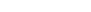, such that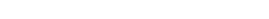. Show that there does not exist any integer b with f(b) = 14.

## Solution:

Consider the auxiliary polynomial g(x) = f(x) - 3. Clearly, according to the problem,  g(x) has four distinct integer roots. Hence we may write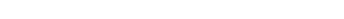where Q(x) is an integer coefficient polynomial. (Since by factor theorem if 't' is a root of a polynomial f(x) then (x-t) is it's factor).

Suppose there exists an integer b such that f(b) = 14; then g(b) = 14-3 =11. Hence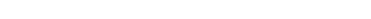.are distinct so are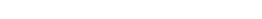. Therefore the equationindicates that 11 can be written as the product of at least 4 different integers which is impossible (as 11 is a prime). Indeed even if we consider -1, 1 and 11 , we have only three integers whose product is 11.

Thus we have a contradiction and proving that there does not exist an integer b such that f(b) 14.

Key Idea: Factor TheoremThis is a Test of Mathematics Solution of Subjective 42 (from ISI Entrance). The book, Test of Mathematics at 10+2 Level is Published by East West Press. This problem book is indispensable for the preparation of I.S.I. B.Stat and B.Math Entrance.

Also Visit: I.S.I & CMI Entrance Course of Cheenta

## Problem

Let f(x) be a polynomial with integer coefficients. Suppose that there exist distinct integers, such that. Show that there does not exist any integer b with f(b) = 14.

## Solution:

Consider the auxiliary polynomial g(x) = f(x) - 3. Clearly, according to the problem,  g(x) has four distinct integer roots. Hence we may writewhere Q(x) is an integer coefficient polynomial. (Since by factor theorem if 't' is a root of a polynomial f(x) then (x-t) is it's factor).

Suppose there exists an integer b such that f(b) = 14; then g(b) = 14-3 =11. Hence.are distinct so are. Therefore the equationindicates that 11 can be written as the product of at least 4 different integers which is impossible (as 11 is a prime). Indeed even if we consider -1, 1 and 11 , we have only three integers whose product is 11.

Thus we have a contradiction and proving that there does not exist an integer b such that f(b) 14.

Key Idea: Factor Theorem

### Knowledge Partner